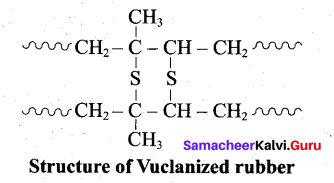# Tamil Nadu 12th Chemistry Model Question Paper 1 English Medium

Students can Download Tamil Nadu 12th Chemistry Model Question Paper 1 English Medium Pdf, Tamil Nadu 12th Chemistry Model Question Papers helps you to revise the complete Tamilnadu State Board New Syllabus and score more marks in your examinations.

## TN State Board 12th Chemistry Model Question Paper 1 English Medium

Instructions:

1. The question paper comprises of four parts
2. You are to attempt all the parts. An internal choice of questions is provided wherever: applicable
3. All questions of Part I, II, III and IV are to be attempted separately
4. Question numbers 1 to 15 in Part I are Multiple choice Questions of one mark each.  These are to be answered by choosing the most suitable answer from the given four alternatives and writing the option code and the corresponding answer
5. Question numbers 16 to 24 in Part II are two-mark questions. These are lo be answered in about one or two sentences.
6. Question numbers 25 to 33 in Part III are three-mark questions. These are lo be answered in about three to five short sentences.
7. Question numbers 34 to 38 in Part IV are five-mark questions. These are lo be answered in detail. Draw diagrams wherever necessary.

Time: 3 Hours
Maximum Marks: 70

Part-I

Answer all the questions. Choose the correct answer. [15 x 1 = 15]

Question 1.
The change in Gibbs free energy for a reaction is expressed by ……………… .
(a) ∆G = ∆H + T∆S
(b) ∆G = ∆H – TS
(c)G = H-TS
(d) ∆G = ∆H – T∆S
(d) ∆G = ∆H – T∆S

Question 2.
Consider the following statements
(i) In interhalogen compounds, the central atom will be the smaller one.
(ii) It can be formed only between two halogen and not more than two halogens.
(iii) They are strong reducing agents.
Which of the above statement(s) is / are not correct?
(a) (i) only
(b) (ii) and (iii)
(c) (i) and (iii)
(d) (iii) only
(c) (i) and (iii)Question 3.
Thermodynamically the most stable form of carbon is ………….
(a) Diamond
(b) graphite
(c) Fullerene
(d) none of these
(b) graphite

Question 4.
Which one of the following transition element has maximum oxidation states?
(a) Manganese
(b) Copper
(c) Scandium
(d) Titanium
(a) ManganeseQuestion 5.
An excess of silver nitrate is added to 100ml of a 0.01M solution of penta aquachlorido chromium (III) chloride. The number of moles of AgCl precipitated would be
(a) 0.02
(b) 0.002
(c) 0.01
(d) 0.2
(b) 0.002

Question 6.
Match the following.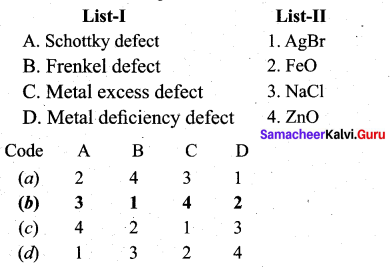Question 7.
If the initial concentration of the reactant is doubled, the time for half reaction is also doubled. Then the order of the reaction is ………..
(a) Zero
(b) one
(c) Fraction
(d) none
For a first order reaction t1/2 is independent of initial concentration.
i.e n ≠ 1; for such cases.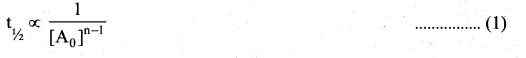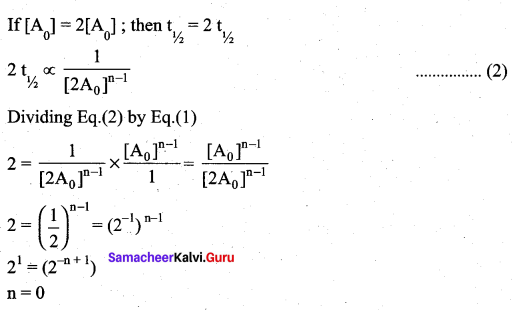Question 8.
Which of the following is not a buffer solution?
(a) CH3COOH + CH3COONa
(b) NH4OH + NH4Cl
(c) H2CO3 + NaHCO3
(d) NaOH + NaCl
(d) NaOH + NaClQuestion 9.
In the electrochemical cell: ZnlZnSO4 (0.01M)||CuSO4 (1.OM)|Cu, the emf of this Daniel cell is E1 When the concentration of ZnSO4 is changed to 1.0M and that CuSO4 changed to 0.01M, the emf changes to E2 From the followings, which one is the relationship between E1 and E2?
(a) E1 < E2
(b) E1 > E2
(c) E2 = 0↑E1
(d) E1 = E2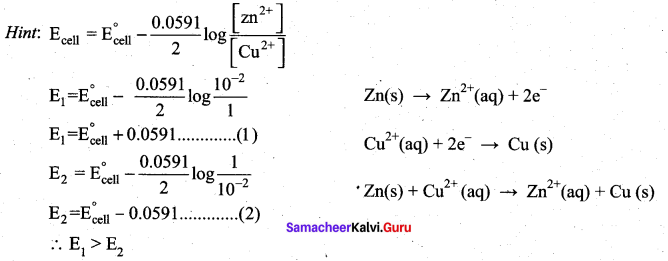(b) E1 > E2

Question 10.
Which method is used to prepare metal sols?
(a) ultrasonic dispersion
(b) mechanical dispersion
(c) Bredig’s arc method
(d) peptisation
(c) Bredig’s arc method

Question 11.
What are the products formed when methoxy ethane is treated with hydroiodic acid?
(a) Phenol + iodomethane
(b) Iodomethane + Ethanol
(c) Iodoethane + Methanol
(d) Iodobenzene + Methane
(b) Iodomethane + Ethanol

Question 12.
Predict the product Z in the following series of reactions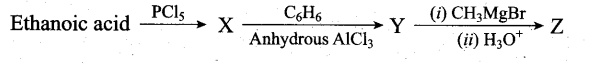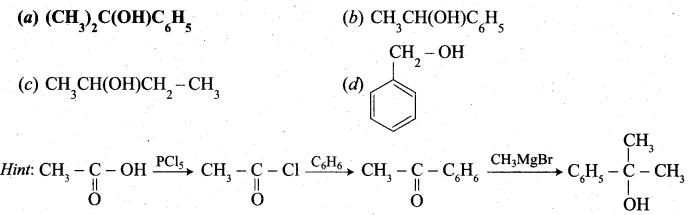(a) (CH3)2C(OH)C6H5

Question 13.
Assertion(A): 2-nitro propane is more acidic than nitro methane.
Reason (R): When the number of alkyl group attached to a carbon increases, acidity decreases, due to +I effect of alkyl groups.
(a) Both A and R are correct but R is not the correct explanation of of A
(b) Both A and R are correct and R is the correct explanation of A
(c) A is correct but R is wrong
(d) A is wrong but R is correct
(d) A is wrong but R is correctQuestion 14.
Glucose(HCN) Product (hydrolysis) Product (HI + Heat) A, the compound A is
(a) Heptanoic acid
(b) 2-Iodohexane
(c) Heptane
(d) Heptanol
(a) Heptanoic acid

Question 15.
Which one of the following is used to provide relief from the allergic effects?
(a) cetrizine
(b) ampicillin
(c) erythromycin
(d) milk of magnesia
(a) cetrizine

Part – II

Answer any six questions. Question No. 21 is compulsory. [6 x 2 = 12]

Question 16.
Magnesite (Magnesium carbonate) is calcined to obtain magnesia, which is used to make refractory bricks. Write the decomposition reaction.
Magnesite is a carbonate of magnesium. Magnesite when heated at 800°C to 1000°C at the CO2 content in it is driven off. The residue so obtained is known as calcined magnesite.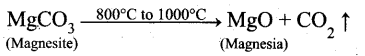Question 17.
Mention the application of Xenon?

• Xenon is used in fluorescent bulbs, flash bulbs and lasers.
• Xenon emits an intense light in discharge tubes instantly. Due to this it is used in high speed electronic flash bulbs used by photographers.

Question 18.
Compare the stability of Ni4+ and Pt4+ from their ionisation enthalpy values.

 lE Ni Pt I 737 864 II 1753 1791 III 3395 2800 IV 5297 4150

(i) Ni4+I.E. = 737+ 1753 + 3395 + 5297= 11182 kJmol-1
(ii) Pt4+ I.E. = 864 + 1791 + 2800 + 4150 = 9605 kJ mol-1
Pt4+ compounds are stable than Ni4+ compounds because the energy needed to remove 4 electrons in Pt is less than that of Ni.Question 19.
Why is glass considered as super cooled liquid?
Glass is an amorphous solid. Like liquids it has a tendency to flow, though very slowly. The proof of this fact is that glass panes in the windows or doors of old buildings are invariably found to be slightly thicker at the bottom than at the top.

Question 20.
Write Arrhenius equation and explains the terms involved.
Arrhenius equation : $$\mathrm{k}=\mathrm{Ae}^{-}\left(\frac{\mathrm{E}_{a}}{\mathrm{RT}}\right)$$
where, k = Rate constant
A = Arrhenius factor (frequency factor)
Ea = Activation energy
R = Gas constant
T = Absolute temperature (in K)

Question 21.
Can Fe3+ oxidises Bromide to bromine under standard conditions?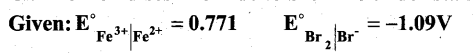Required half cell reaction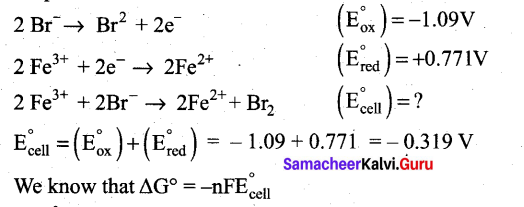If E°cell is -ve; ∆G is +ve and the cell reaction is non-spontaneous.
Hence, Fe3+ cannot oxidise Bromide to Bromine.

Question 22.
What are used of Urotropine? Give its structure.
This is the structure of Urotropine (Hexamethylene tetramine)

• Urotropine is used as a medicine to treat urinary infection.
• Nitration of Urotropine under controlled condition gives an explosive RDX (Research and development explosive). It is also called cyclonite or cyclotri methylene trinitramine.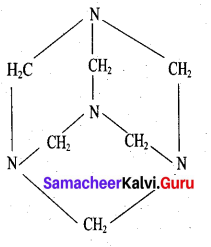Question 23.
Ethylamine is soluble in water whereas aniline is not. Give reason.
Ethylamine when added to water forms intermolecular H-bonds with water. And therefore it is soluble in water. But aniline does not form H-bond with water to a very large extent due to the presence of a large hydrophobic – C H group. Hence, aniline is insoluble in water.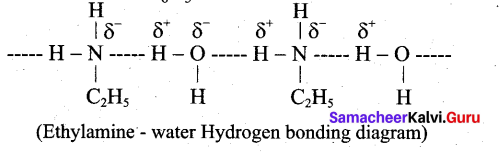Question 24.
What are antihistamines? Give example and mention its use.

• Antihistamines block histamine release from histamine – 1 receptors.
• It is used to provide relief from the allergic effects.
•Part – III

Answer any six questions. Question No. 31 is compulsory. [6 x 3 = 18]

Question 25.
Explain the action of heat on boric acid.
Boric acid when heated at 373 K gives metaboric acid and at 413 K, it gives tetraboric acid. When heated at red hot, it gives boric anhydride which is a glassy mass.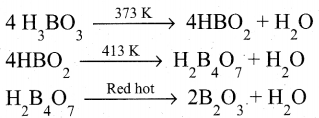Question 26.
Draw the structure of (a) white phosphorous (b) red phosphorous
(a) White phosphorous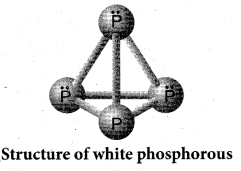(b) Red phosphorous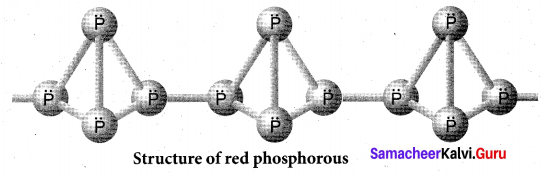Question 27.
Why do zirconium and Hafnium exhibit similar properties?

1. The element of second and third transition series resemble each other more closely than the elements of first and second transition series due to lanthanoid contraction.
2. e.g., Zr- 4d series -Atomic radius 145 pm
Hf — 5d series – Atomic radius 144 pm
3. The radii are very similar even though the number of electrons increases.
4. Zr and Hf have very similar chemical behaviour, having closely similar radii and electronic configuration.
5. radius dependent properties such as lattice energy, solvation energy are similar.
6. Thus lanthanides contraction leads to formation of pair of elements and those known as chemical twins, e.g., Zr – Hf

Question 28.
How will derive the formula of density of a unit cell?
Using the edge length of a unit cell, we can calculate the density (p) of the crystal by considering a cubic unit cell as follows.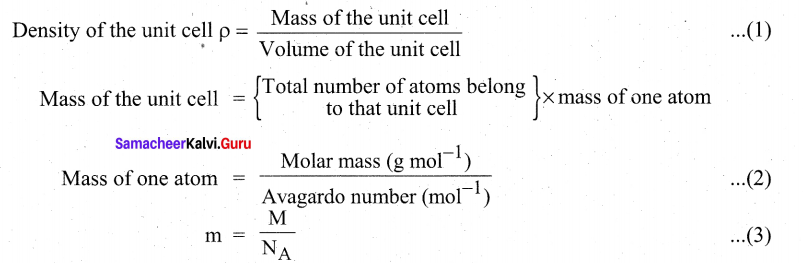Substitute the value (3) in (2)
Mass of the unit cell = $$n \times \frac{\mathbf{M}}{N_{\mathrm{A}}}$$
For a cubic unit cell, all the edge lengths are equal, i.e., a = b = c
Volume of the unit cell = a x a x a = a3
∴ Density of the unit cell = $$\rho=\frac{n M}{a^{3} N_{A}}$$

Question 29.
The salt of strong acid and strong base does not undergo hydrolysis. Explain.
(i) In this case, neither the cations nor the anions undergo hydrolysis. Therefore the solution remains neutral.
(ii) For example, in the aqueous solution of NaCl, its ions Na+ and Cl ions have no tendency to react with H+ or OH ions of water. This is because the possible products of such reaction are NaOH and HC1 which are completely dissociated. As a result, there is no change in the concentration of H+ and OH ions and hence the solution continues to remain neutral.Question 30.
Write a note about medicinal applications of colloids.

• Antibodies such as penicillin and streptomycin are produced in colloidal form for suitable injections. They cure pneumonia.
• Colloidal gold and colloidal calcium are used as tonics.
• Milk of magnesia is used for stomach troubles.
• Silver sol protected by gelatine known as Argyrol is used as eye lotion.

Question 31.
Predict the major product, when 2-methyl but -2-ene is converted into an alcohol in each of the following methods.
(i) Acid catalysed hydration (ii) Hydroboration (iii) Hydroxylation using bayers reagent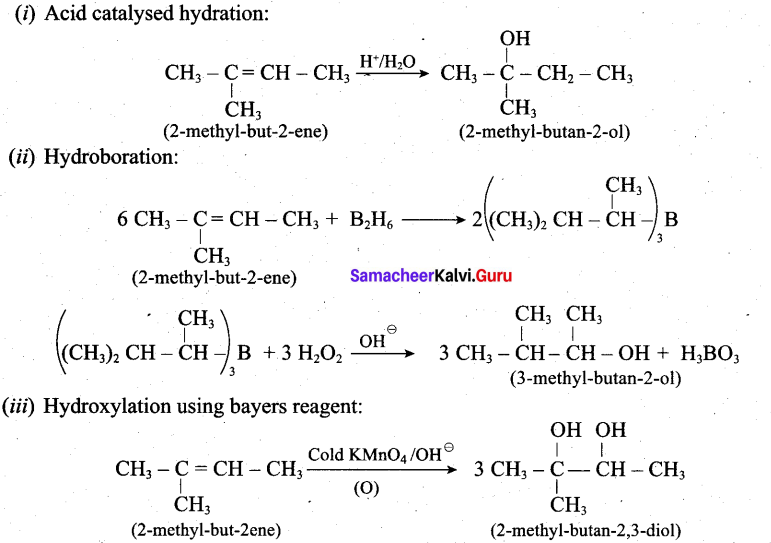Question 32.
Write the reactions of (i) aromatic and (ii) aliphatic primary amines with nitrous acid.
Aromatic primary amines react with HNO2 at 273-278 K to form aromatic diazonium salts.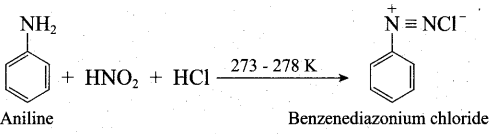Aliphatic primary amines also react with HNO2 at 273-278 K to form aliphatic diazonium salts. But these are unstable even at low temperature and thus decomposes readily to form a mixture of compounds consisting of alkyl chlorides, alkenes and alcohols, out of which ‘ alcohols generally predominates.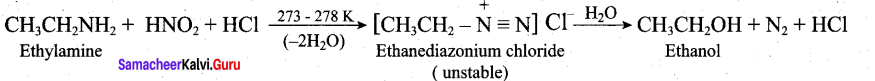Question 33.

• Uses of preservatives reduce the product spoilage and extend the shelf-life of food.
• Addition of vitamins and minerals reduces the mall nutrient.
• Flavouring agents enhance the aroma of the food.
• Antidxidants prevent the formation of potentially toxic oxidation products of lipids and other food constituents.

Part – IV

Answer all the questions. [5 x 5 = 25]

Question 34.
(a) (i) Out of coke and CO, which is better reducing agent for the reduction of ZnO? Why? (2)
(ii) An element (A) extracted from kernite. A reacts with nitrogen at high temperature gives B. A reacts with alkali to form C. Find out A, B and C. Give the chemical equations. (3)
[OR]
(b) (i) Deduce the oxidation number of oxygen in hypofluorous acid – HOF. (2)
(ii) Which catalyst is used in the conversion of acetaldehyde to acetic acid? Give equation. (3)
(a) (i) Coke (C) is a better reducing agent for the reduction of ZnO.
Because, when we use coke, the reduction can be easily carried out at 673 K. Thus Carbon (Coke) reduces zinc oxide more easily than carbon monoxide (CO). From the Ellingham diagrams, it is quite clear that the reduction of zinc oxide is more favourable using coke (∆G for the formation of carbon monoxide from carbon is more negative).

(ii)

• An element (A) extracted from kernite is boron.
• Boron reacts with nitrogen at high temperature to give. Boron nitride (B)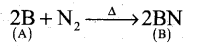• Boron reacts with alkali (NaOH) to give sodium borate (C).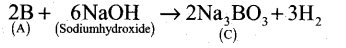A Boron B B Boron nitride BN C Sodium borate Na3BO3

(b) (i)

•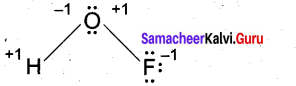[OR]

In case of O – F bond is HOF, fluorine is most electronegative element. So its oxidation number is -1. Thereby oxidation number of O is +1. Similarly in case of O – H bond is HOF. O is highly electronegative than H. So its oxidation number is -1 and oxidation number of H is +1. So, Net oxidation of oxygen is – 1 + 1 = 0.
(ii)

•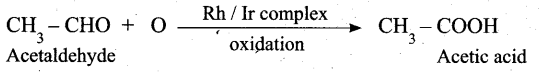Question 35.
(a) (i) Prove that H2SO4 is a strong dibasic acid. (2)
(ii) Distinguish tetrahedral and octahedral voids. (3)
[OR]
(b) In an octahedral crystal field, draw the figure to show splitting of d orbitals. (5)
(a) (i) Sulphuric acid forms two types of salts namely sulphates and bisulphates.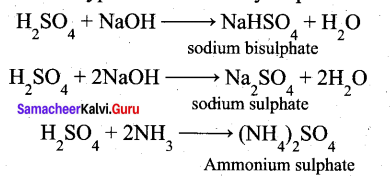(ii)

 Tetrahedral void Octahedral void 1. A single triangular void in a crystal is surrounded by four (4) spheres and is called a tetrahedral void A double triangular void like c is  surrounded by six(6) spheres and is called an octahedral void 2. A sphere of second layer is above the void of the first layer, a tetrahedral void is formed The voids ¡n the first layer are partially covered by the spheres of layer now such a void is called a octahedral void 3. This constitutes four spheres, three in the lower and one in upper layer. When the centres of these four spheres are joined a tetrahedron is formed This constitutes six spheres, three in the lower layer and three in the upper layer. When the centers of these six spheres are joined an octahedron is formed 4. The radius of the sphere which can be accommodated in an octahedral hole without disturbing the structure should not exceed 0.414 times that of the structure forming sphere The sphere which can be placed in  a tetrahedral hole without disturbing the close packed structure should not have a radius larger than 0.225 times the radius of the sphere.forming the structure 5. Radius of an tetrahedral void r/R = 0.225 Radius of a octahedral void r/R = 0.414

[OR]

(b) Step 1: In an isolated gaseous state, all the five d orbitals of the central metal ion are degenerate.
Initially, the ligands form a spherical field of negative charge around the metal. In this filed, the energies of all the five d orbitals will increase due to the repulsion between the electrons of the metal and the ligand.

Step 2: The ligands are approaching the metal atom in actual bond directions. To illustrate this let us consider an octahedral field, in which the central metal ion is located at the origin and the six ligands are coming from the +x, -x, +y, -y,
+z and -z directions as shown below.

As shown in the figure, the orbitals lying along the axes dx2 – y2 and dz2 orbitals will experience strong repulsion and raise in energy to a greater extent than the orbitals with lobes directed between the axes (dxy, dyz and dzx). Thus the degenerate d orbitals now split into two sets and the process is called crystal field splitting.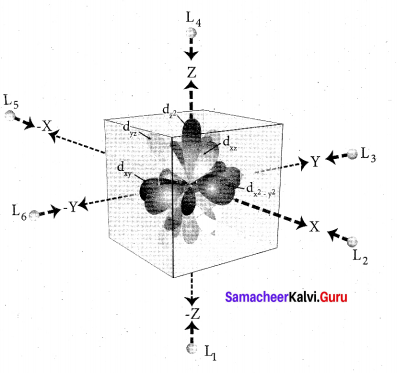Step 3: Up to this point the complex formation would not be favoured. However, when the ligands approach further, there will be an attraction between the negatively charged electron and the positively charged metal ion, that results in a net decrease in energy. This decrease in energy is the driving force for the complex formation.
During crystal field splitting in octahedral field, in order to maintain the average energy of the orbitals (barycentre) constant, the energy of the orbitals dx2 y2 and d z2 (represented as e2 orbitals) = will increase by 3/5 ∆0 while that of the- other three orbitais dxy , dyz and dzx (represented t2gas t orbitais) decrease by 2/5 ∆0 . Here, ∆0 represents the crystal field splitting energy in the octahedral field.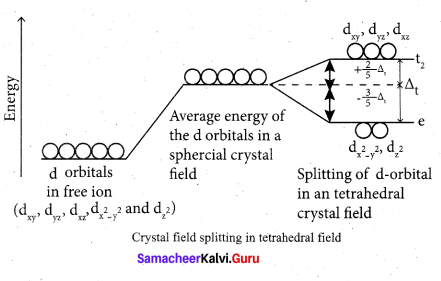Question 36.
(a) Explain briefly the collision theory of bimolecular reactions. (5)
[OR]
(b) Discuss about the hydrolysis of salt of weak acid and weak base and derive pH value for the solution. (5)
(a) Collision theory is based on the kinetic theory of gases. According to this theory, chemical reactions occur as a result of collisions between the reacting molecules. Let its understand this theory by considering the following reaction.
A2(g) + B2(g) → 2AB(g)

If we consider that, the reaction between A2 and B2 molecules proceeds through collisions between them, then the rate would be proportional to the number of collisions per second.
Rate ∝ Number of molecules colliding per litre per second (or) Rate ∝ Collision rate.
The number of collisions is directly proportional to the concentration of both A2 and B2.
Collision rate ∝ [A2][B2] ; Collision rate = Z [A2][B2] where, Z is a constant.

The collision rate in gases can be calculated from kinetic theory of gases. For a gas at room temperature (298K) and 1 atm pressure, each molecule undergoes approximately 109 collisions per second, i.e., 1 collision in 10-9 second. Thus, if every collision resulted in reaction, the reaction would be complete in 10-9 second. In actual practice this does not happen. It implies that all collisions are not effective to lead to the reaction.

In order to react, the colliding molecules must possess a minimum energy called activation energy.
The molecules that collide with less energy than activation energy will remain intact and no reaction occurs.

Fraction of effective collisions (f) is given by the following expression, $$\mathbf{f}=e^{\frac{-\mathbf{E}_{\mathrm{a}}}{\mathrm{RT}}}$$

Fraction of collisions is further reduced due to orientation factor i.e., even if the reactant collide with sufficient energy, they will not react unless the orientation of the reactant molecules is suitable for the formation of the transition state.

The fraction of effective collisions (f) having proper orientation is given by the steric factor R ’ Rate = P x f x collision rate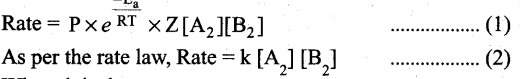Where k is the rate constant.
On comparing equation (1) and (2), the rate constant k is,
k = PZ$$\frac{-E_{a}}{R T}$$

[OR]

(b) i. Consider the hydrolysis of ammonium acetate
CH3COONH4(aq) CH3COO(aq) + NH+4(aq)

ii. In this case both the cation (NH4+ ) and (CH3COO) anion have the tendency to react with water.
CH3COO+H2 ⇌ CH3COOH + OH
NH4+ + H2O ⇌ NH4OH + H+

iii. The nature of the solution depends on the strength of acid (or) base i.e., if Ka > Kb, then the solution is acidic and pH < 7, if Ka < Kb then the solution is basic and pH > 7.
If Ka= Kb, then the solution is neutral.
iv. The relation between the dissociation constant Ka, Kb and hydrolysis constant is given
Ka. Kb .Kh = Kw
v. pH of the solution pH = 7 + 1/2 PKa – 1/2 pKbQuestion 37.
(a) (i) Ionic conductance at infinite dilution of Al3+and SO42- are 189 and 160 mho cm2 equiv-1 . Calculate the equivalent and molar conductance of the electrolyte Al2(SO4)3 at infinite dilution. (3)
(ii) Suggest a way to determine λ0m value of water (2)

(OR)

(b) (i) Why does bleeding stop by rubbing moist alum. (2)
(ii) How is glycerol reacts with fuming nitric acid? (or) How would you convert glycerol into nitroglycerine? (3)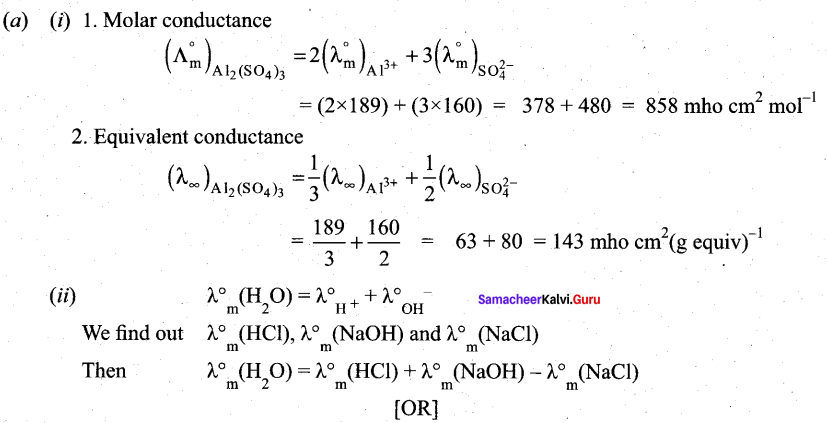(b) (i) Blood is a colloidal sol. When we rub the injured part with moist alum then coagulation of blood takes place. Hence main reason is coagulation, which stops the bleeding. Therefore bleeding stop by rubbing moist alum.
(ii)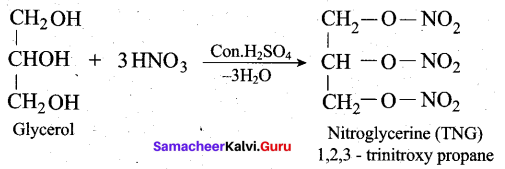Question 38.
(a) (i) What is crossed cannizaro reaction? Explain it. (2)
(ii) Complete the following reactions.(3)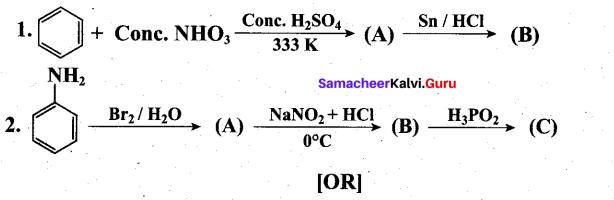(b) (i) Is the following sugar, D – sugar or L – sugar? (2)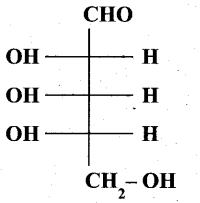(ii) Write a note on vulcanization of rubber. (3)
(a) (i) When Cannizaro reaction (Auto redox reaction) takes place between two different aldehyde, the reaction is called as crossed cannizaro reaction.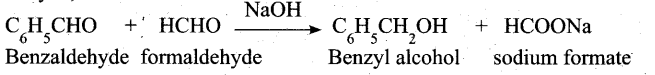In crossed cannizaro reaction more reactive aldehyde is oxidized and less reactive aldehyde is reduced.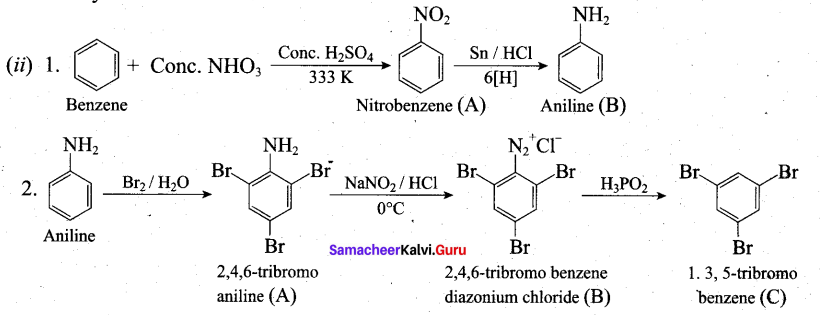[OR]

(b) (i) L-Sugar
(ii)

• Natural rubber is very soft and brisky. it has high water absorption capacity and low tensile strength. Its properties can be improved by a process called vulcanization.
• Natural rubber is mixed with 3-5% sulphur and heated at 100-150°C causes cross linking of the cis – 1, 4 polyisoprene chains through disulphide (-S-S-) bonds.
• The physical properties of rubber can be altered by controlling the amount of sulphur that is used for vulcanization. When 3 to 10% sulphur is used the resultant rubber is somewhat harder but flexible.

Following properties of rubber improved by vulcanization:

• Tensile strength
• Elasticity
• Hardness
• Tear strength
• Resistance to solvants.